# Differential expression (OMICS) in Excel tutorial

2017-03-03

This tutorial will show you how to set up and interpret a differential expression analysis in Excel using the XLSTAT statistical software.

## Dataset for running differential expression analysis in XLSTAT

For this tutorial we use a simulated data table corresponding to 36 biological samples of diseased and healthy individuals belonging to three different genotypes. For each sample, the expression of 1561 genes is measured through RNA quantification.

RNAs are stored in rows and samples in columns. A genotype factor and a health status factor are added to the right of the data matrix. Factors row numbers correspond to the number of samples (number of columns of the data matrix).

An Excel sheet with both the data and the results can be downloaded by clicking here.

## Goal of this tutorial

The aim of this tutorial is to use the differential expression tool in XLSTAT to identify differentially expressed genes according to two factors: genotype (three levels: BB, BK, KK) and health status (two levels: healthy and diseased). For each factor, we will:

1)     Perform non-specific filtering to eliminate features which very low variability.

2)     Perform classic one-way ANOVAs automatically on each of all of the remaining features and extract p-values.

3)     Correct p-values using appropriate methods to avoid detecting significant effects by chance.

Features (genes represented by RNAs) associated to the lowest p-values are those which are the most significantly affected by the studied factor. This tool is very useful to detect pools of genes which are linked to a disease for example.

For more than two-level factors (e.g. genotype), we will be able to perform multiple pairwise comparisons per feature.

For two-level factors (e.g. health status), we will be able to generate volcano plots to visualize both the statistical and biological significances associated to all features.

Notice that the differential expression tool in XLSTAT may also be used to study the effects of explanatory variables on protein production or metabolite regulation in an OMICs high-throughput data context.

## Differential expression in XLSTAT: setting up the analysis

To perform a differential expression analysis, click on XLSTAT-OMICs / Differential expression. In the General tab, select the data matrix in the Features/individuals table field. Here, the individuals are represented by our samples. You do not need to change the features in rows option, as genes are stored in rows in the dataset. It is mandatory to select the first column in the dataset containing feature IDs. XLSTAT needs this information to let the user identify interesting features with their names in the analysis’ output. In the explanatory variables field, select the two columns containing the affiliation of every sample to factor levels.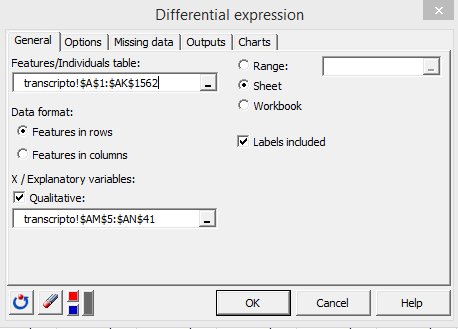In the Options tab, select a Parametric test type. This option will produce one one-way ANOVA per factor and feature. For small sample numbers, we recommend using the non-parametric method instead, which replaces one-way ANOVAs by Kruskal-Wallis tests. In the Post hoc corrections, choose the Benjamini-Hochberg procedure, which is very commonly used in differential expression studies. It is part of the False Discovery Rate ( FDR ) p-value corrections family. It is well suited for studies involving the computation of a large number of p-values as it is  less stringent than corrections which are part of the Family Wise Error Rate ( FWER ) family, such as the Bonferroni correction. Set the number of low p-values to keep to 30, to avoid displaying huge lists of p-values in the output (high p-values are not quite interesting in the context of our study). Activate the multiple pairwise comparisons option and choose Tukey (HSD) in order to obtain pairwise multiple comparisons among the genotype levels for each gene. Finally, activate the non-specific filtering option, choose %(Std. dev.) with a 50% threshold to eliminate 50% of genes based on the lowest standard deviations criterion prior to analyses.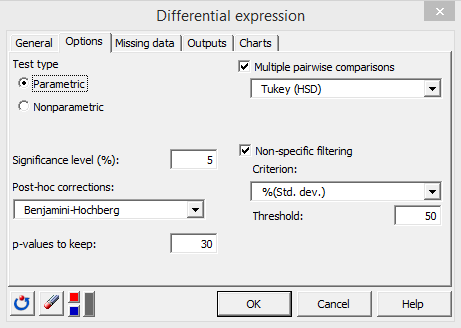In the Charts tab, activate both the Histogram of p-values and the volcano plot options.

The two following options represent two ways of representing biological effects on the volcano plot’s x axis. We will choose the Log2(means ratio), because our data are not transformed. Activate the Identify features option. XLSTAT will therefore use a special color for highly significant features at both the statistical and the biological scales, and according to the two following thresholds. Choose 1 for Threshold(x). A log2(means ratio) of 1 means that the mean on the numerator is twice as high as the mean at the denominator. Inversely, a log2(means ratio) of -1 means that the mean on the denominator is twice as high as the mean of the numerator. A log2(means ratio) of 2 or -2 represents a fold change of 2², and so on. Choose a 0.001 p-value threshold in the Threshold(y) box. This means that the statistical significance threshold will be –log10(0.001).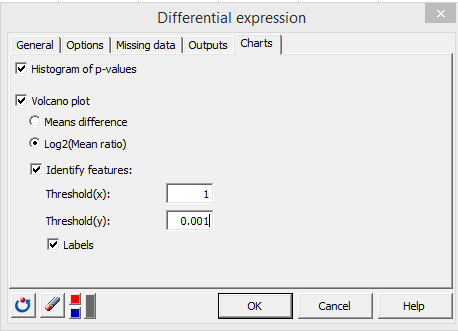Click on the OK button.

## Differential expression in XLSTAT: interpreting the results

After a summary about different options used in the analysis, the number of features which were eliminated by non-specific filtering is displayed. Then one analysis is displayed per factor.

First, a table displaying the 30 most significant features sorted according to increasing p-values is displayed. The table contains feature name, penalized p-values, significance, and RNA quantity means for each factor level. If a p-value is significant, the user may be interested in multiple pairwise comparisons represented by letters associated to means. Two levels sharing the same letter are not significantly different. Two levels having no letter in common are significantly different.

For the genotype factor, there are no significant p-value at alpha = 0.05. In this case, interpreting multiple comparisons is not relevant for any of the features.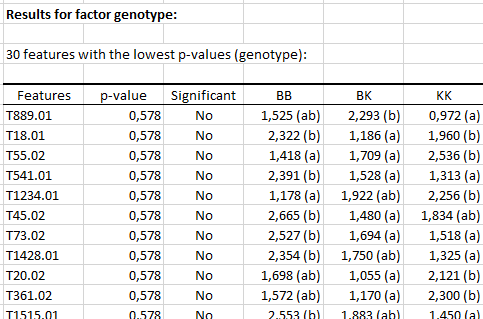The p-values histogram shows that p-values are homogeneously distributed.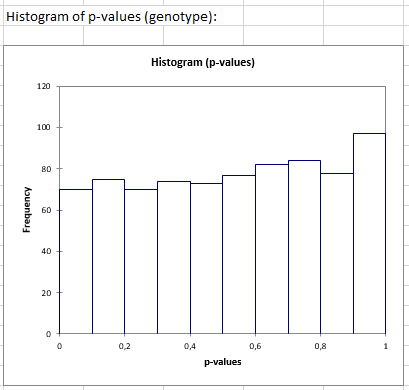The healthy or diseased factor seems to affect the expression of two genes: T1157.01 and T106.02. The first one has a higher expression in healthy samples, and the second one has a higher expression in the diseased samples.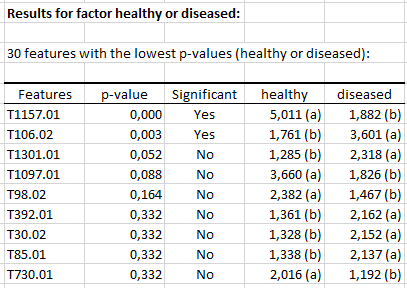Both features can be visualized on the volcano plot: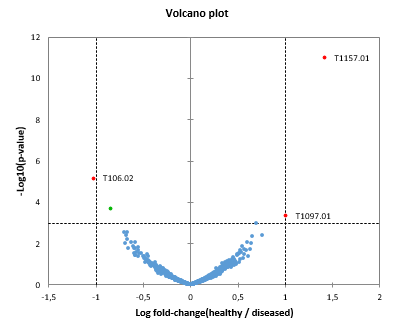Features located at the top-left and top-right corners of the chart are labeled. They correspond to the features that overtake biological and statistical significance thresholds (dashed lines).

Notice that the p-values used to compute the –log10(p-values) on the volcano plot are the raw, uncorrected p-values.

#### Contact our technical support team: support@xlstat.com

https://cdn.desk.com/
false
desk